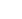# Binomial Logistic Regression/ Simple Logistic Regression

This is used to predicts if an observation falls into one of categories of dichotomous dependent variables based one or more dependent variables

1. Click Analyze- Regression- Binary Logistic

-the logistic Regression dialogue box opens

1. Transfer the dependent variable into the dependent box and independent variables into the Covariates box.
• For standard logistic regression, you should ignore the Previous and the Next buttons because they are for sequential (hierarchical) logistic regression.
• The Method: Option needs to be kept at the default value which is “ENTER”
• The enter method is the name given by SPSS statistics to standard regression analysis
1. Click the Categorical button.

-the Logistic Regression: Define Categorical Variables dialogue box

– SPSS statistic requires you to define all the categorical predictor values in the logistic regression model.

1. Transfer the Independent, categorical variable from the Covariates box to the Categorical Covariates: box.
2. In the Change Contrast area, Change the Reference Category from the Last Option to First Option. Then click the Change Button.
• Whether you choose Last or First will depend on how you set up your data.
1. Click the Continue button.